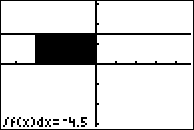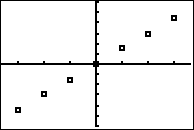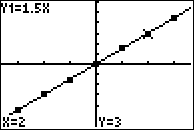••• ##### Device
• TI-83 Plus Family
• TI-84 Plus
• TI-84 Plus Silver Edition
•TI-84 Plus C Silver Edition
•TI-84 Plus CE

# Calculus: Accumulation Functions

by Texas Instruments#### Overview

In this activity, students examine functions defined by a definite integral. They create tables and graphs for integration functions. They understand the foundation of the Fundamental Theorem of Calculus.

#### Key Steps

•Students will create tables and graphs for functions of in the form of a definite integral.

•Students will use the graphing handheld to produce a scatter plot.

•Students will understand the foundation of the Fundamental Theorem of Calculus.